Like   Tweet   Pin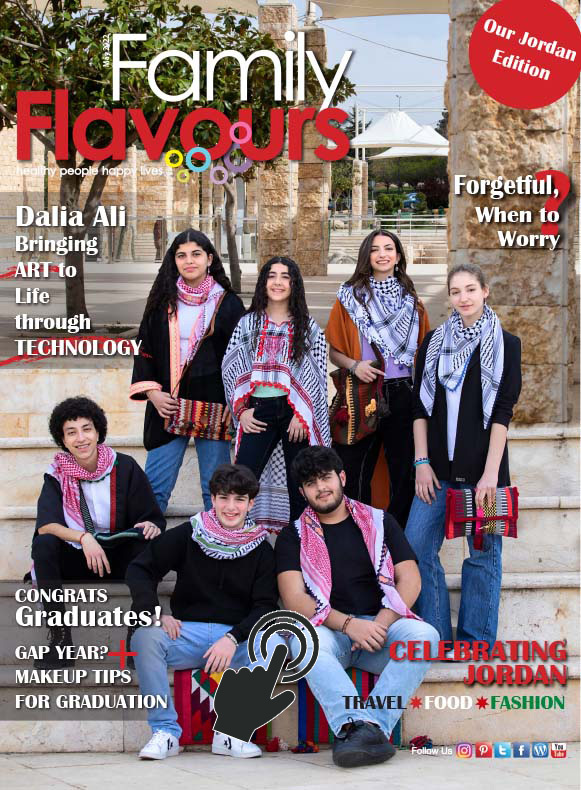Cover photo by Portrait Photographer Anastasia Casey

Welcome to Our Jordan Edition!
Click on the photo for a sneak peek of our May 2022 issue

## In Our Issue:

▪ Dalia Ali Bringing ART to Life through TECHNOLOGY
 ▪ Dalia Ali Bringing ART to Life through TECHNOLOGY

▪ Forgetful When to Worry?
 ▪ Forgetful When to Worry?

▪ JORDAN: Travel, Food, Fashion
 ▪ JORDAN: Travel, Food, Fashion
 table div table+table+table div table{width:100%;padding:0}table div table+table+table div table img{width:96.23%;padding:0;float:none}table div table+table+table div table td{width:100%;padding:0 1.88% 18px}We thank you for supporting us for almost two decades. From cover to cover, Family Flavours and Nakahat Ailiyeh, our sister publication, are produced in Jordan with over 30 experts contributing to our print and digital platforms as well as our training workshops and awareness raising events.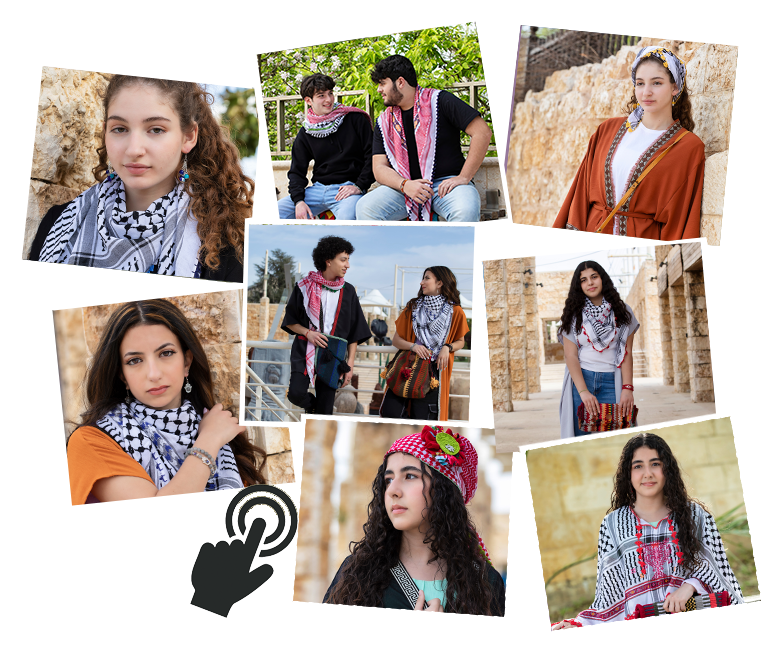Click on photos to see our photo gallery
 In our May 2022 edition, our teen fashionistas help us support local vendors, thanks to our partnership with Virtual Bazaar; they engage and showcase Jordan-inspired fashion.
 table div table+table+table+table+table+table+table div table{width:100%;padding:0}table div table+table+table+table+table+table+table div table img{width:96.23%;padding:0;float:none}table div table+table+table+table+table+table+table div table td{width:100%;padding:0 1.88% 18px}German photographer, Miriam Wozny, commemorates Jordan’s Independence Day by sharing her experience and photos of exploring Wadi Rum on horseback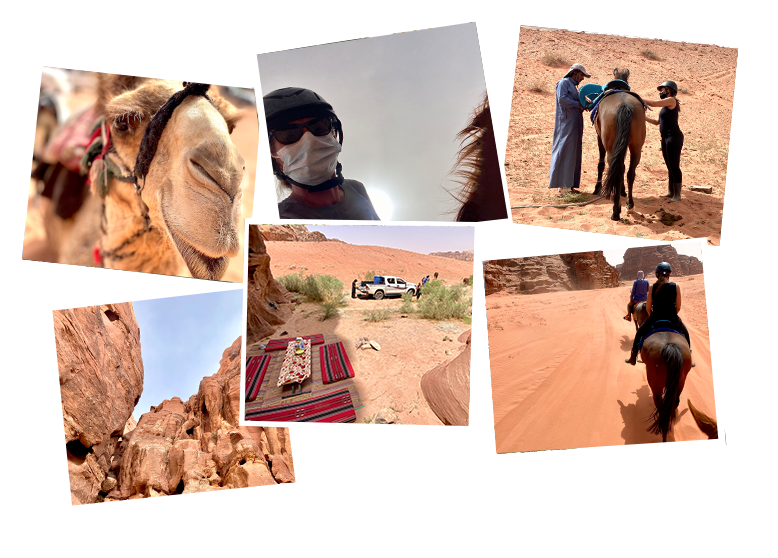table div table+table+table+table+table+table+table+table+table+table div table{width:100%;padding:0}table div table+table+table+table+table+table+table+table+table+table div table img{width:96.23%;padding:0;float:none}table div table+table+table+table+table+table+table+table+table+table div table td{width:100%;padding:0 1.88% 18px}Celebrating Jordan with a sweet twist: Banah Sogayar celebrates Jordan’s Independence Day (25 May) with sweet layers designating the colours of the Jordanian flag-get inspired!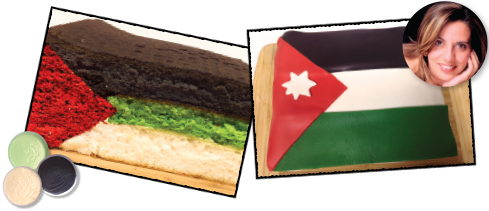table div table+table+table+table+table+table+table+table+table+table+table+table+table div table{width:100%;padding:0}table div table+table+table+table+table+table+table+table+table+table+table+table+table div table img{width:96.23%;padding:0;float:none}table div table+table+table+table+table+table+table+table+table+table+table+table+table div table td{width:100%;padding:0 1.88% 18px}## A Recipe for Success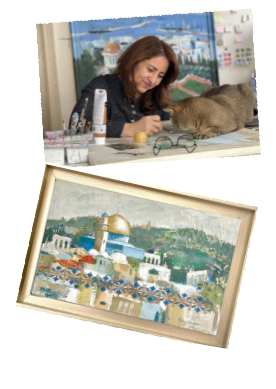Technology meets art through Dalia Ali’s latest exhibit in Amman. She chats with us and explains how she connects to Palestine- a place she’s never been to. It’s difficult to believe with the depth and passion she brings to her work!

 table div table+table+table+table+table+table+table+table+table+table+table+table+table+table+table+table div table{width:100%;padding:0}table div table+table+table+table+table+table+table+table+table+table+table+table+table+table+table+table div table img{width:96.23%;padding:0;float:none}table div table+table+table+table+table+table+table+table+table+table+table+table+table+table+table+table div table td{width:100%;padding:0 1.88% 18px}Graduation doesn’t necessarily mean your teen is ready to jump straight into university. Educational Psychologist Dina Halaseh highlights the benefits of a Gap Year and other possible options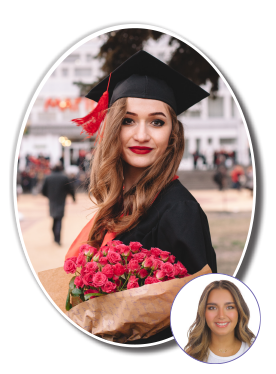Your teen can be confident on stage with 2022 Graduation Makeup Looks, brought to you by our Makeup Artist Rama Hijazi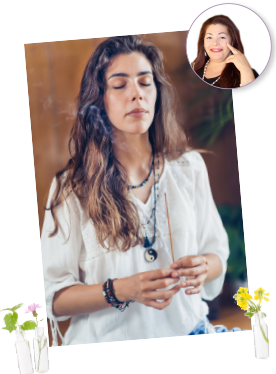In this issue, Aromatherapist and Cosmetologist Zenab Ishtay shares tips for successfully getting through allergy season

 table div table+table+table+table+table+table+table+table+table+table+table+table+table+table+table+table+table+table+table+table+table div table{width:100%;padding:0}table div table+table+table+table+table+table+table+table+table+table+table+table+table+table+table+table+table+table+table+table+table div table img{width:96.23%;padding:0;float:none}table div table+table+table+table+table+table+table+table+table+table+table+table+table+table+table+table+table+table+table+table+table div table td{width:100%;padding:0 1.88% 18px}## Partners in Health Awareness

 table div table+table+table+table+table+table+table+table+table+table+table+table+table+table+table+table+table+table+table+table+table+table+table div table,table.module-22{width:49.81%;float:left;padding:0}table div table+table+table+table+table+table+table+table+table+table+table+table+table+table+table+table+table+table+table+table+table+table+table div table a{border:0 none;text-decoration:none}table div table+table+table+table+table+table+table+table+table+table+table+table+table+table+table+table+table+table+table+table+table+table+table div table img{width:100%!important;border:0 none;text-decoration:none}table div table+table+table+table+table+table+table+table+table+table+table+table+table+table+table+table+table+table+table+table+table+table+table div table td{width:100%;padding:0 20px 20px 0} Thanks to our partnership with Al ‘Oun for Alzheimer's Patient Care Association, we can help raise awareness and bring support to families in Jordan impacted by Alzheimer’s. In this edition, Consultant Neurologist Dr Marina Hadidi helps us understand the difference between normal ageing and the early signs of Alzheimer's
 table div table+table+table+table+table+table+table+table+table+table+table+table+table+table+table+table+table+table+table+table+table+table+table+table div table{width:100%;padding:0}table div table+table+table+table+table+table+table+table+table+table+table+table+table+table+table+table+table+table+table+table+table+table+table+table div table img{width:96.23%;padding:0;float:none}table div table+table+table+table+table+table+table+table+table+table+table+table+table+table+table+table+table+table+table+table+table+table+table+table div table td{width:100%;padding:0 1.88% 18px}## Our News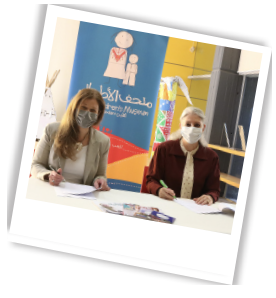With a focus on educational programmes and collaborative events that
promote safe spaces for learning through play, the Children’s Museum Jordan renews a two-year partnership agreement with Family Flavours and Nakahat ‘Ailiyeh parenting magazines. The cooperation agreement is based on a 14-year partnership serving children and families in Jordan.

Ambassadors of Family Health & Wellbeing programme is a new initiative that engages our experts from across fields, in the Museum’s activities to raise awareness in areas that promote health and learning through play

 table div table+table+table+table+table+table+table+table+table+table+table+table+table+table+table+table+table+table+table+table+table+table+table+table+table+table+table div table{width:100%;padding:0}table div table+table+table+table+table+table+table+table+table+table+table+table+table+table+table+table+table+table+table+table+table+table+table+table+table+table+table div table img{width:96.23%;padding:0;float:none}table div table+table+table+table+table+table+table+table+table+table+table+table+table+table+table+table+table+table+table+table+table+table+table+table+table+table+table div table td{width:100%;padding:0 1.88% 18px}## Partner with Us!Interested in partnering with us for future events? Contact us at 0796551718

 table div table+table+table+table+table+table+table+table+table+table+table+table+table+table+table+table+table+table+table+table+table+table+table+table+table+table+table+table+table+table div table{width:100%;padding:0}table div table+table+table+table+table+table+table+table+table+table+table+table+table+table+table+table+table+table+table+table+table+table+table+table+table+table+table+table+table+table div table img{width:96.23%;padding:0;float:none}table div table+table+table+table+table+table+table+table+table+table+table+table+table+table+table+table+table+table+table+table+table+table+table+table+table+table+table+table+table+table div table td{width:100%;padding:0 1.88% 18px}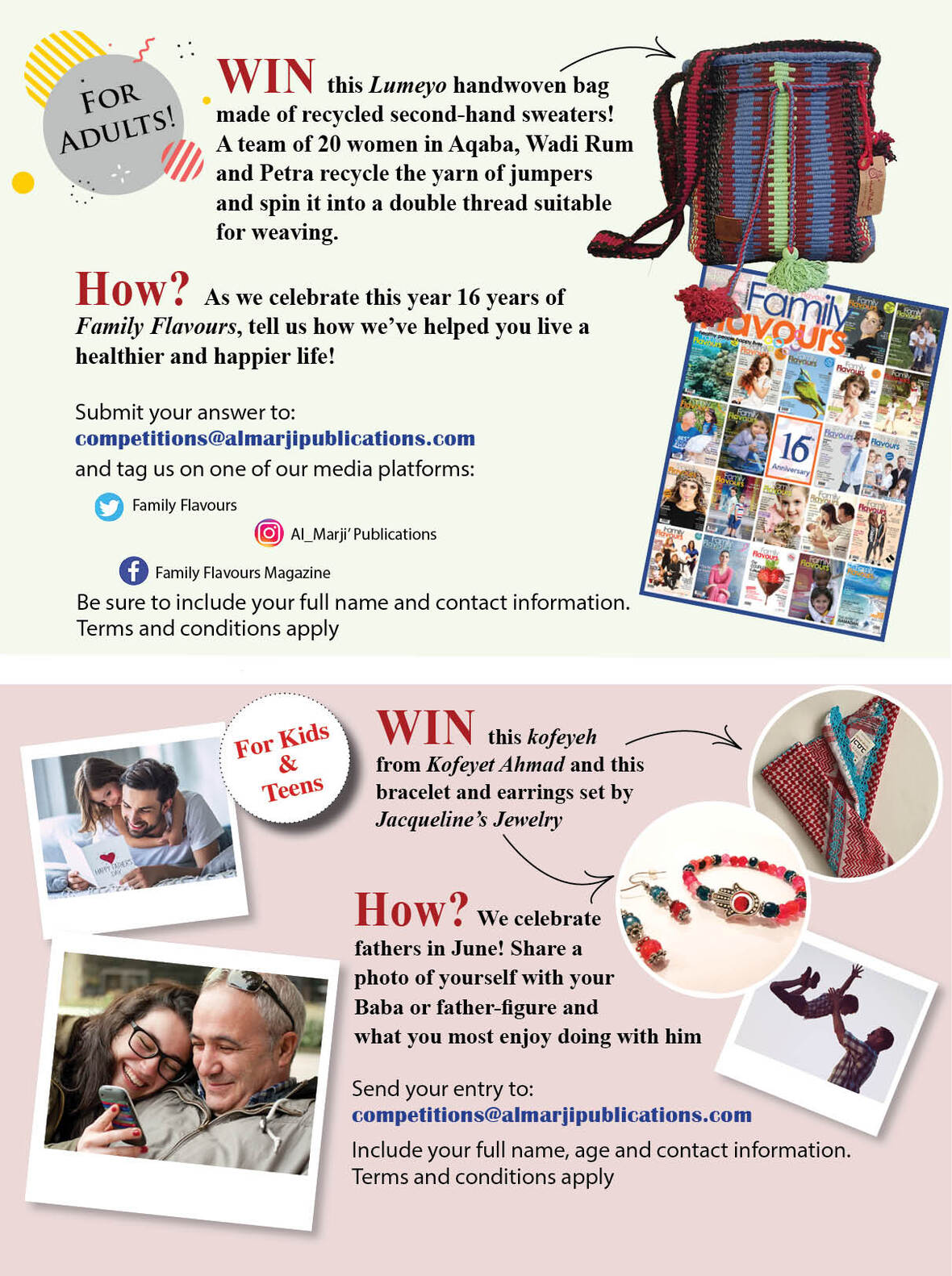table div table+table+table+table+table+table+table+table+table+table+table+table+table+table+table+table+table+table+table+table+table+table+table+table+table+table+table+table+table+table+table+table+table div table{width:100%;padding:0}table div table+table+table+table+table+table+table+table+table+table+table+table+table+table+table+table+table+table+table+table+table+table+table+table+table+table+table+table+table+table+table+table+table div table img{width:96.23%;padding:0;float:none}table div table+table+table+table+table+table+table+table+table+table+table+table+table+table+table+table+table+table+table+table+table+table+table+table+table+table+table+table+table+table+table+table+table div table td{width:100%;padding:0 1.88% 18px}Get Family Flavours delivered directly to your inbox!
Send us an email ourmailbag@almarjipublications.com